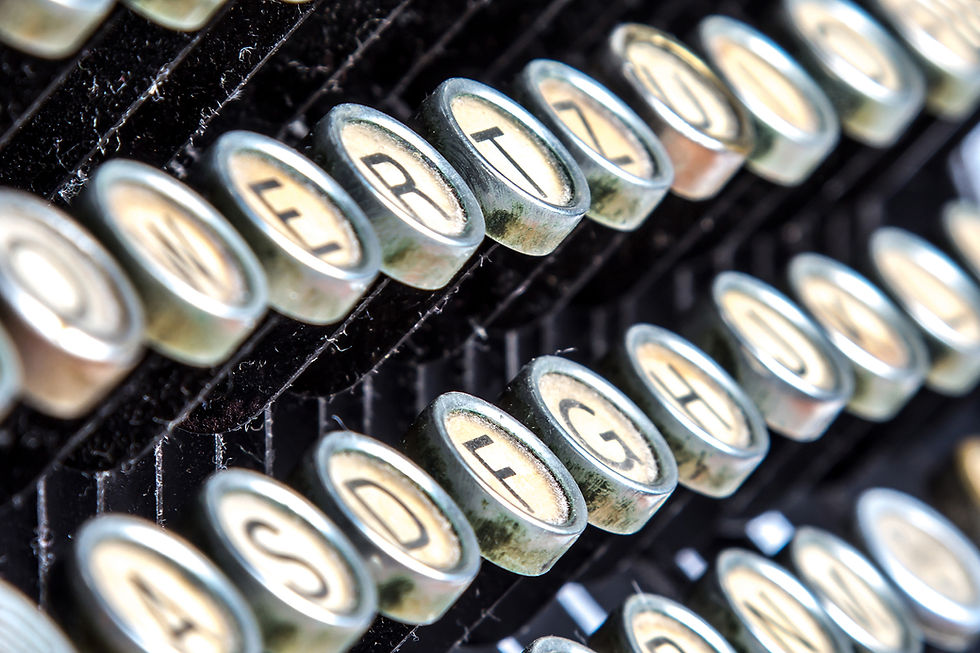top of page

# How to pass the key pressed to the function when using onkeypress in Python's turtle modulePython's turtle module is a brilliant tool to use for teaching coding. One of the main functions used when creating interactive animations or games is onkeypress() which enables the binding of a keypress with a function.

If you've spent enough time playing with this module, you have probably found yourself asking the following question: How do I pass the information about which key has been pressed to the function that is being called when the key is pressed?

Here's how onkeypress() is used:

```import turtle

window = turtle.Screen()
fred = turtle.Turtle()

def draw_square():
for _ in range(4):
fred.forward(100)
fred.left(90)

window.onkeypress(draw_square, 'space')
window.listen()

turtle.done()```

You've created an instance of the Screen() and the Turtle() objects, defined a function that draws a square and then used onkeypress() to bind the space bar key to this function.

So when you run the program and press space, the turtle will draw a square.

What if you want to draw a polygon with any number of sides. Let's limit the number of sides to a maximum of nine for this example.

The function draw_square() can be changed to draw_polygon(n_sides):

```def draw_polygon(n_sides):
for _ in range(n_sides):
fred.forward(400 / n_sides)
fred.left(360 / n_sides)```

What you'd love to be able to do now is to bind the key '5' on the keyboard with

draw_polygon(5) and the key '7' with draw_polygon(7) and so on.

Except that you cannot.

The function you use in the call to onkeypress() cannot take any input arguments. To put this another way, the key used in onkeypress() will trigger the function, but the information about which key has been pressed is lost and cannot be passed on to the function.

Of course we can create lots of different functions and keybindings, for example a function called draw_triangle() bound to the key '3', draw_square() bound to '4' and so on. But life's too short and we don't like these repetitive patterns when we code.

So let's fix this. There must be a hack to overcome this limitation.

Let's return to the fact that the function used in the call to onkeypress() cannot have any input arguments. We cannot write window.onkeypress(draw_polygon(4), '4') as this would simply run the draw_polygon(4) function when the onkeypress() function is executed, drawing the square right away, and then bind whatever is returned by the function to the key '4'. In this case the function returns None so nothing will happen when the key '4' is pressed.

This must mean that the function used as the first argument of onkeypress() must be defined without any input parameters, right?

Not quite, no.

Let's have a look at the following modification:

`window.onkeypress(lambda n=4: draw_polygon(n), '4')`

We're now using an anonymous lambda function as the first parameter in onkeypress(). However we have defined the lambda function so that its own input parameter, n, has a default value. This means the function can be called without an input argument, as required by any function used within onkeypress().

This means that when the key '4' is pressed, the lambda function is called with no input arguments, but as n defaults to 4, then draw_polygon(4) is called, and we have a square.

Why is this useful? Let's extend this idea a bit further:

```import turtle

window = turtle.Screen()
fred = turtle.Turtle()

def draw_polygon(n_sides):
fred.clear()
for _ in range(n_sides):
fred.forward(400 / n_sides)
fred.left(360 / n_sides)

for n in range(3, 10):
window.onkeypress(lambda n=n: draw_polygon(n), str(n))

window.listen()

turtle.done()```

The keys '3' through to '9' have now been bound to lambda functions that will return the draw_polygon() function with the required input argument. Press '3' and you get a triangle, press '5' and you get a pentagon, and so on.

We have managed to pass the information about which key has been pressed to the function we want to execute.

Edit (17/04/21): An alternative to using the lambda function, suggested by Mike Driscoll, is to use functools.partial which allows you to create a version of a function with some of its input arguments pre-assigned. After importing functools, the line in which the key bindings are being made can be replaced with:

```window.onkeypress(functools.partial(draw_polygon, n_sides=n),
str(n),
)```

And here's another example to simulate a typewriter using turtle:

```import turtle
import string

window = turtle.Screen()
window.tracer(0)

text = turtle.Turtle()
text.hideturtle()
text.penup()

left_margin = -200
top_margin = 200
line_spacing = 50

text.setposition(left_margin, top_margin)

font = ("Courier", 25)

def write(key):
text.write(key, font=font)
text.forward(font*0.65)

def carriage_return():
text.sety(text.ycor() - line_spacing)
text.setx(left_margin)

all_characters = string.ascii_letters + string.punctuation + string.whitespace
for key in all_characters:
window.onkeypress(lambda key=key: write(key), key)

window.onkeypress(carriage_return, "Return")
window.listen()

turtle.done()

```

So if you ever find yourself writing lots of repetitive functions and binding each one of them to a different key on the keyboard, check whether the lambda function can come to the rescue!

Enjoyed this and want to learn more Python for free? Check out our sister site The Python Coding Book, for in-depth guides on all things Python, or follow us on Twitter @codetoday_ and @s_gruppetta_ct